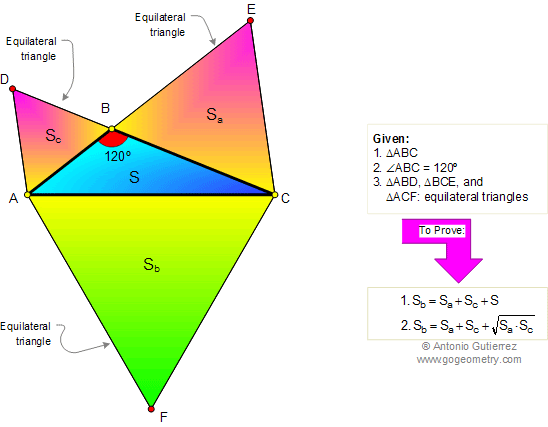## Wednesday, December 24, 2008

### Elearn Geometry Problem 212: 120 Degree Triangle, AreasSee complete Problem 212 at:
gogeometry.com/problem/p212_triangle_120_equilateral_area.htm

120 degrees triangle, equilateral triangle, areas. Level: High School, SAT Prep, College geometry

#### 1 comment:

1.Sa= (root3)*a^2/4
Sb= (root3)*b^2/4
Sc= (root3)*c^2/4
S= 1/2*ac*Sin 120=(root3)*ac/4
but by cosine rule
b^2=a^2+c^2+ac so ac= b^2- a^2- c^2
s=(root3)/4*ac
= (root3)/4[b^2-c^2-a^2]
= Sb-Sc-Sa
hence Sb=Sa+Sc+S -----(1)
but S^2=Sa*Sc
S= root of (Sa*Sc)
substitute in equation 1
we get Sb=Sa+Sc+ root of (Sc*Sa)
hence solved.